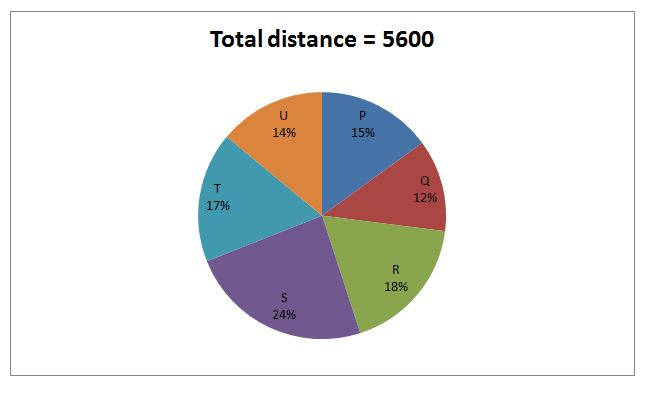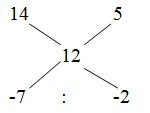# LIC AAO Mains Quantitative Aptitude Questions 2019 (Day-10)

Dear Aspirants, Our IBPS Guide team is providing new series of Quantitative Aptitude Questions for LIC AAO Mains 2019 so the aspirants can practice it on a daily basis. These questions are framed by our skilled experts after understanding your needs thoroughly. Aspirants can practice these new series questions daily to familiarize with the exact exam pattern and make your preparation effective.

[WpProQuiz 6271]

### Click Here for Mains 2019 High-Quality Mocks Exactly on SBI Standard

1) Sanjay invested Rs. 72000 in two different parts, one at 10% CI (Compounded half yearly) and another one at 15% SI. At the end of the year, he received total interest Rs. 9375, then find the amount invested in SI?

a) Rs. 28000

b) Rs. 42000

c) Rs. 36000

d) Rs. 25000

e) None of these

2) B is 5/4 times as efficient as A. If A can fill the 3/5 of the tank in 15 min, what fraction of the capacity of the tank would remain incomplete if B can fill the tank independently for 10 min only?

a) 2/3

b) 1/3

c) ¼

d) ½

e) None of these

3) Kavin’s present age is four times her son’s present age and two fifth of her father’s present age. The average present age of all of them is 40 years. Find the sum of Kavin’s son’s present age and Kavin’s father’s present age?

a) 92 years

b) 74 years

c) 88 years

d) 86 years

e) None of these

4) The Average marks obtained by 45 students in an examination is 12. If the average marks of passed students are 14 and that of failed students are 5, then find the number of students who passed the examination?

a) 35

b) 42

c) 30

d) 26

e) None of these

5) Aravind, Ganesh and Thyagu started a business by investing Rs. 24000, Rs. 30000 and Rs. 36000 respectively. Arvind is a working partner and Ganesh and Thyagu are sleeping partner in a business. For managing the business, Aravind receives 10 % of profit. Find the difference between the share of Aravind and Thyagu, if the total profit at the end of the year is Rs. 72000?

a) Rs. 2520

b) Rs. 1850

c) Rs. 1440

d) Rs. 2890

e) None of these

Directions (Q. 6 – 10): Study the following information carefully and answer the given questions.

The following pie chart shows the total distance covered by 6 different persons.6) P travels for 60 Km per hour. After travelling for 2 hour, P triples his speed. Then find the total time taken by him to reach the destination?

a) 4 hours

b) 5 hours

c) 7 hours

d) 6 hours

e) None of these

7) U travels on 56 km/hr to reach the place on time. After travelling for 2 hours, he realized that he had missed the important document in the starting point and returns back to the place and take the document and begin his travelling with increased the speed and reached the destination on time. How much speed increased by him?

a) 22.4 Km/hr

b) 18.6 Km/hr

c) 24.8 Km/hr

d) 20.2 Km/hr

e) None of these

8) If S travels at the speed of 56 km/hr and 64 km/hr in first and second hour respectively and reached the destination in 19 hours, then find the speed to cover the remaining distance?

a) 78 Km/hr

b) 84 Km/hr

c) 72 Km/hr

d) 66 Km/hr

e) None of these

9) Find the difference between the total distance covered by P and R together to that of total distance covered by T and U together?

a) 104 Km

b) 112 Km

c) 96 Km

d) 88 Km

e) None of these

10) Q travels 240 km by flight, 156 km by train and remaining distance by car. The speed of flight and train is 160 km/hr, 78 km/hr respectively. Altogether taken by him to reach the destination is 7 ½ hours. Find the speed of the car?

a) 75 Km/hr

b) 64 Km/hr

c) 72 Km/hr

d) 69 Km/hr

e) None of these

Let us take one part be x and another one be 72000 – x,

According to the question,

x*[(1 + 10/200)2 – 1)] + [(72000 – x)*15*1]/100 = 9375

x*[(210/200)2 – 1)] + 10800 – (15x/100) = 9375

(41x/400) + 10800 – (15x/100) = 9375

(41x/400) – (15x/100) = 9375 – 10800

-19x/400 = -1425

x = 1425*(400/19) = 30000

The amount invested in S.I = 72000 – x = 72000 – 30000 = Rs. 42000

Efficiency ratio of A and B = 4 : 5

Day ratio = > A : B = 5 : 4

A can fill the 3/5 of the tank in 15 min

= > (3/5)*work = 15 min

= > Whole work = 25 min

A takes 25 min to fill the tank, So, B takes,

= > 5’s = 25

= > 1’s = 5

B can fill the tank in, 20 min

B can fill the tank independently for 10 min only.

B’s 10 min work = 10/20 = 1/2

Remaining = 1 – 1/2 = ½

Half of the tank will remain incomplete.

The ratio of present age of Kavin and her son’s age = 4 : 1 (4x, x)

Kavin’s present age = (2/5)* Kavin’s father’s present age

Kavin’s father’s present age = 10x

According to the question,

x + 4x + 10x = 120

15x = 120

x = 120/15

x = 8

Kavin’s father’s present age = 10x = 80 years

Let the number of passed students be x,

45*12 = 14x + (45 – x)*5

540 = 14x + 225 – 5x

540 – 225 = 9x

9x = 315

X = 35

Total number of passed students = 35

= > 7 : 2

9’s = 45

1’s = 5

Total number of passed students = 7’s = 35

Shortcut:= > 7 : 2

9’s = 45

1’s = 5

Total number of passed students = 7’s = 35

= > 72000*(10/100) = Rs. 7200

Remaining = 72000 – 7200 = Rs. 64800

The ratio of investment of Aravind, Ganesh and Thyagu

= > 24000 : 30000 : 36000

= > 4 : 5 : 6

15’s = 64800

1’s = 4320

The share of Aravind = (4*4320) + 7200 = Rs. 24480

The share of Thyagu = (6*4320) = Rs. 25920

Required difference = 25920 – 24480 = Rs. 1440

Directions (6-10):

= > 72000*(10/100) = Rs. 7200

Remaining = 72000 – 7200 = Rs. 64800

The ratio of investment of Aravind, Ganesh and Thyagu

= > 24000 : 30000 : 36000

= > 4 : 5 : 6

15’s = 64800

1’s = 4320

The share of Aravind = (4*4320) + 7200 = Rs. 24480

The share of Thyagu = (6*4320) = Rs. 25920

Required difference = 25920 – 24480 = Rs. 1440

Total distance covered by P = 5600*(15/100) = 840 Km

P travels 60 km per day. After 2 days he reached (60*2) = 120 km.

Remaining distance = 840 – 120 = 720 Km

Required time = 2 + (720/180) = 2 + 4 = 6 hours

Total distance travelled by U = 5600*(14/100) = 784 Km

Time = (784/56) = 14 hours

Total hours taken by him to collect the document = 2 + 2 = 4 hours

Remaining time = 14 – 4 = 10 hours

Required answer = (784/10) = 78.4 km/hr

Increased speed = 78.4 – 56 = 22.4 Km/hr

Total distance covered by S = 5600*(24/100) = 1344 Km

The distance covered by him in 2 hours = 56 + 64 = 120 Km

Remaining distance = 1344 – 120 = 1224 Km

Required speed = (1224/17) = 72 Km/hr

The total distance covered by P and R together

= > 5600*(33/100) = 1848 Km

The total distance covered by T and U together

= > 5600*(31/100) = 1736 Km

Required difference = 1848 – 1736 = 112 Km

Total distance covered by Q = 5600*(12/100) = 672 Km

Let the speed of the car be x,

According to the question,

(240/160) + (156/78) + (276/x) = 15/2

(3/2) + 2 + (276/x) = (15/2)

(276/x) = (15/2) – (7/2)

(276/x) = 4

X = (276/4) = 69 Km/hr

Speed of the car = 69 Km/hr